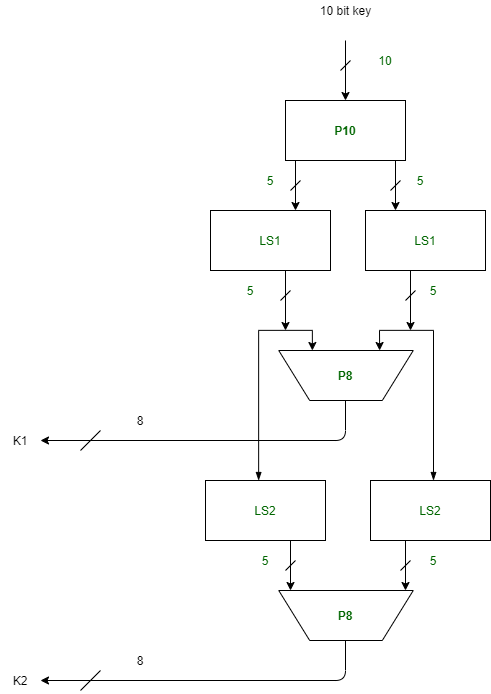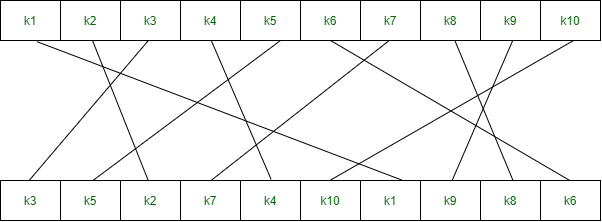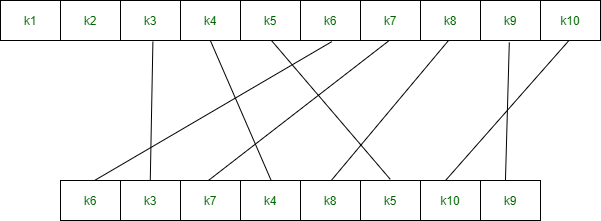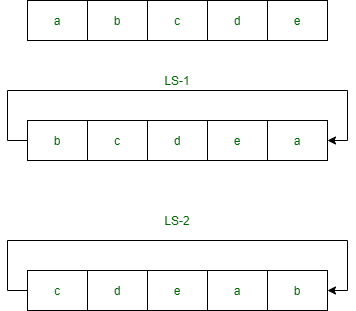# Simplified Data Encryption Standard Key Generation

• Last Updated : 27 Sep, 2021

Simplified Data Encryption Standard (S-DES) is a simple version of the DES Algorithm. It is similar to the DES algorithm but is a smaller algorithm and has fewer parameters than DES. It was made for educational purposes so that understanding DES would become simpler.  It is a block cipher that takes a block of plain text and converts it into ciphertext.  It takes a block of 8 bit.

It is a symmetric key cipher i.e. they use the same key for both encryption and decryption. In this article, we are going to demonstrate key generation for s-des encryption and decryption algorithm. We take a random 10-bit key and produce two 8-bit keys which will be used for encryption and decryption.

Attention reader! Don’t stop learning now. Get hold of all the important CS Theory concepts for SDE interviews with the CS Theory Course at a student-friendly price and become industry ready.

Key Generation Concept: In the key generation algorithm, we accept the 10-bit key and convert it into two 8 bit keys. This key is shared between both sender and receiver.In the key generation, we use three functions:

1. Permutation P102. Permutation P83. Left ShiftStep 1: We accepted a 10-bit key and permuted the bits by putting them in the P10 table.

```Key = 1 0 1 0 0 0 0 0 1 0
(k1, k2, k3, k4, k5, k6, k7, k8, k9, k10) = (1, 0, 1, 0, 0, 0, 0, 0, 1, 0)

P10 Permutation is: P10(k1, k2, k3, k4, k5, k6, k7, k8, k9, k10) = (k3, k5, k2, k7, k4, k10, k1, k9, k8, k6)
After P10, we get 1 0 0 0 0 0 1 1 0 0```

Step 2: We divide the key into 2 halves of 5-bit each.

`l=1 0 0 0 0, r=0 1 1 0 0`

Step 3: Now we apply one bit left-shift on each key.

`l = 0 0 0 0 1, r = 1 1 0 0 0 `

Step 4: Combine both keys after step 3 and permute the bits by putting them in the P8 table. The output of the given table is the first key K1.

```After LS-1 combined, we get 0 0 0 0 1 1 1 0 0 0
P8 permutation is: P8(k1, k2, k3, k4, k5, k6, k7, k8, k9, k10) = (k6, k3, k7, k4, k8, k5, k10, k9)
After P8, we get Key-1 : 1 0 1 0 0 1 0 0```

Step 5: The output obtained from step 3 i.e. 2 halves after one bit left shift should again undergo the process of two-bit left shift.

```Step 3 output - l = 0 0 0 0 1, r = 1 1 0 0 0
After two bit shift - l = 0 0 1 0 0, r = 0 0 0 1 1```

Step 6: Combine the 2 halves obtained from step 5 and permute them by putting them in the P8 table. The output of the given table is the second key K2.

```After LS-2 combined = 0 0 1 0 0 0 0 0 1 1
P8 permutation is: P8(k1, k2, k3, k4, k5, k6, k7, k8, k9, k10) = (k6, k3, k7, k4, k8, k5, k10, k9)
After P8, we get Key-2 : 0 1 0 0 0 0 1 1```

Final Output:

```Key-1 is: 1 0 1 0 0 1 0 0
Key-2 is: 0 1 0 0 0 0 1 1```
My Personal Notes arrow_drop_up